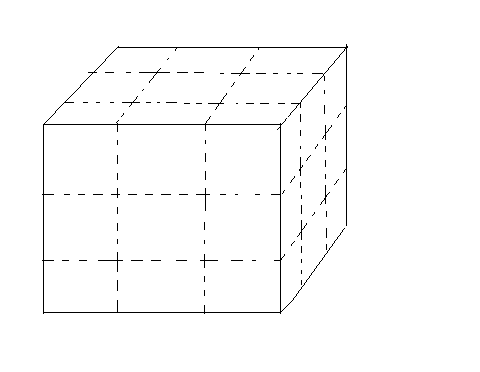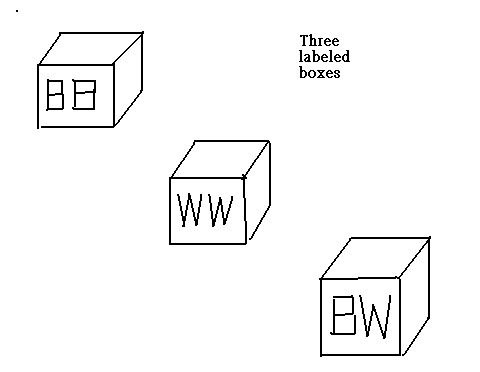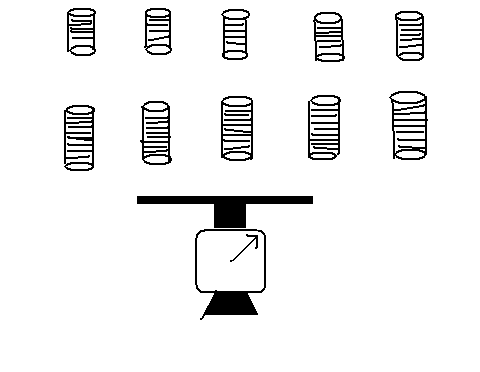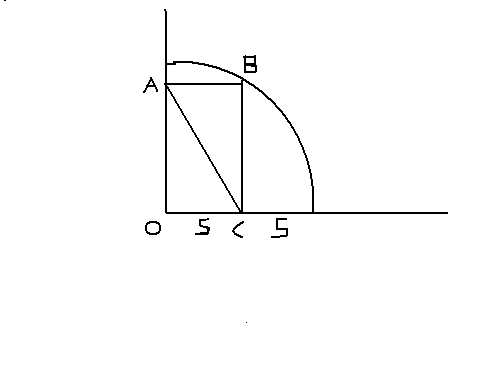Puzzles

--------------------------------------------------------------------------------------------------------------------------------------------------------------------------------

1. Chessboard problem.

The props for this problem are a chessboard and 32 dominoes. Each domino is of such size that it exactly covers two adjacent squares on the board. The 32 dominoes therefore can cover all 64 of the chessboard squares. But now suppose we cut off two squares diagonally opposite corners of the board and discard one of the dominoes. Is it possible to place the 31 dominoes on the board so that all the remaining 62 squares are covered? If so, show how it can be done. If not, prove it impossible.

(Hint: The key to this problem is that each domino covers exactly two squares of different colour.)-------------------------------------------------------------------------------------------------------------------------------------------------------------------------------

2. Cutting the cube.

A carpenter, working with a buzz saw, wishes to cut a wooden cube, three inches on a side, into 27 one-inch cubes. He can do this easily by making six cuts through the cube, keeping the pieces together in the cube shape. Can he reduce the number of necessary cuts by rearranging the pieces after each cut?

(The reader is encouraged to work it out physically. The solution is a bit tricky. )-------------------------------------------------------------------------------------------------------------------------------------------------------------------------------

3. A fork in the road.

A logician vacationing in the South Seas finds himself on an island inhabited by the two proverbial tribes of liars and truth-tellers. Members of one tribe always tell the truth, members of the other always lie. He comes to a fork in a road and has to ask a native bystander which branch he should take to reach a village. He has no way of telling whether the native is a truth-teller or a liar. The logician thinks a moment, then asks one question only. From the reply he knows which road to take. What questions does he ask?

(This is a twist on an old type of logic puzzle)-------------------------------------------------------------------------------------------------------------------------------------------------------------------------------

4. Scrambled Box Tops.

Imagine that you have three boxes. One containing two black marbles, one containing two white marbles, and the third, one black marble and one white marble. The boxes were labeled for their contents---BB, BW, WW---but someone switched the labels so that every box is now incorrectly labeled. You are allowed to take one marble at a time out of any box, without looking inside, and by this process of sampling you are to determine the contents of all three boxes. What is the smallest number of drawings needed to do this?---------------------------------------------------------------------------------------------------------------------------------------------------------------------------------

5. Weigh the coins

You have 10 stacks of coins, each consisting of 10 half-dollars. One entire stack is counterfeit, but you do not know which one. You do know the weight of a genuine half-dollar and you are also told that each counterfeit coin weighs one gram more than it should. You may weigh the coins on a pointer scale. What is the smallest number of weighings necessary to determine which stack is counterfeit.

(A number of clever coin-weighing or ball-weighing problems have aroused widespread interest. Here's a new and simple variation)------------------------------------------------------------------------------------------------------------------------------------------------------------------------------

6. Find the diagonal

A rectangle is inscribed in the quadrant of a circle as shown. Given the unit distances indicated in the picture below. The radius of this quarter of circle is 10 units. The length of OC is 5 units. Find the length of diagonal AC of the rectangle ABCO. As this problem is not that difficult, for the sake of challenge, the time limit is 1 minute.